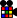# Difference between revisions of "Template:OCLL"

## Contents

### OLL 27

 Name: S, Sune Used in: OCLL, OLL Optimal moves: 7 HTMOLL R U R' U R U2 R'OLL y' R' U2 R U R' U ROLL y L' U2 L U L' U LOLL y2 L U L' U L U2 L'OLL R U' L' U R' U' LOLL U2 r2 U R' U' L' U R U' r' (x')

### OLL 26

 Name: -S, Antisune Used in: OCLL, OLL Optimal moves: 7 HTMOLL y2 R' U' R U' R' U2 ROLL y' R U2 R' U' R U' R'OLL y' L U2 L' U' L U' L'OLL L' U' L U' L' U2 LOLL L' U R U' L U R'OLL y' L' U R U' L U R'OLL y' R U2 R' U2' R' F R2 U' R' U' R U R' F'

### OLL 21

 Name: H, Double Sune Used in: OCLL, OLL Optimal moves: 11 HTM, 10 STMOLL R U R' U R U' R' U R U2 R'OLL y R U2 R' U' R U R' U' R U' R'OLL y F (R U R' U') (R U R' U') (R U R' U') F'OLL y F' (L' U' L U) (L' U' L U) (L' U' L U) FOLL R' U' R U' R' U R U' R' U2 ROLL L U L' U L U' L' U L U2 L'OLL y L' U2 L U L' U' L U L' U LOLL y (R' U R U) (R' U R U') (R' U R U') R' U' R U2 R' U ROLL L' U2 M' (y) R U2 R U2 R' U2 (y') l

### OLL 22

 Name: pi, Bruno, wheel, T-shirt Used in: OCLL, OLL Optimal moves: 9 HTMOLL R U2 R2' U' R2 U' R2' U2 ROLL f (R U R' U') f' F (R U R' U') F'OLL y2 f' (L' U' L U) f F' (L' U' L U) FOLL y2 L' U2 L2 U L2 U L2 U2 L'OLL R U2 R2 U' R' U R U' R U' R' y M' U2 MOLL R' U L U' R U' L' U' L U' L'OLL y2 L U' R' U L' U R U R' U ROLL y2 L' U R U' L U' R' U' R U' R'OLL R U' L' U R' U L U L' U LOLL R U R' U R U2 R' y' R U R' U R U2 R'

### OLL 23

 Name: U, Headlights, Superman Used in: OCLL, OLL Optimal moves: 9 HTMOLL R2 D' R U2 R' D R U2 ROLL L2 D L' U2 L D' L' U2 L'OLL R' U2 R F U' R' U' R U F'OLL l2 U' R D2 R' U R D2 ROLL l2' U' z' U R2 U' L U R2 z' LOLL y' R U R' U' (R U' R' U2)(R U' R' U2) R U R'OLL y2 R' F2 R U2 R U2 R' F2 R U2 R'OLL y2 R2 D R' U2 R D' R' U2 R'OLL (R' U' R U) R' U2 R2 U (R' U R U2 R)OLL R2' B2 R F2' R' B2 R F2' ROLL U2 R2' D R' U2 R D' R' U2 R'OLL R d' R' U R d R' (y) R' U2 ROLL R' U L' U R U' L U2 R' U ROLL (x) R' B2' (R U' R' U) B2' (U' R U)

### OLL 24

 Name: T, chameleon, shark, Hammerhead Used in: OCLL, OLL Optimal moves: 8 HTMOLL r U R' U' L' U R U' x'OLL r U R' U' r' F R F'OLL y' F R U R' U' R U' R' U' R U R' F'OLL R U R2' U' R2 U' R2' U2' R U' R U' R'OLL y' R U' R' (U2 R U R') (U2 R U R') (U R U' R')OLL y x D' R' U R D R' U' R x'OLL y x D L U' L' D' L U L' x'OLL y x' U' R' D R U R' D' R xOLL y2 R' F' r U R U' r' FOLL y2 l' U' L U R U' r' FOLL y' l U l' F R B' R' F'

### OLL 25

 Name: L, Bowtie, Triple-Sune, Side-winder Used in: OCLL, OLL Optimal moves: 8 HTMOLL y2 R' F R B' R' F' R BOLL y2 F R' F' L F R F' L'OLL y' F' r U R' U' r' F ROLL y' x U R' U' L U R U' r'OLL R' F' L' F R F' L FOLL y R' U' (R U R' F') (R U R' U') (R' F R2)OLL y2 R U2' R D R' U2 R D' R2'OLL y2 F R' F' r U R U' r'OLL y2 l' U R D' R' U' R D x'OLL y2 x' L' U' R' U L U' R UOLL x' D R U R' D' R U' l'OLL x' U L' U' R U L U' l'OLL x' R' D R U' R' D' R U xOLL x R' U R D' R' U' R D x'OLL y2 x L U' L' D L U L' D' x'OLL l' U' L' U R U' L U x'OLL U r U R U' L' U R' U' x'OLL R U2 R' U' R U R' U' R U R' U' R U' R'OLL R U R' U R U' R' U R U' R' U R U2 R'OLL y2 L' R U R' U' L U R U' R'OLL F R B R' F' l U' l'OLL U R U2 R' L' U' L U' R U' R' L' U2 L